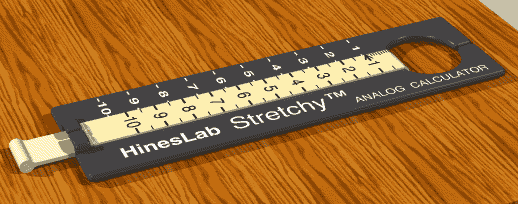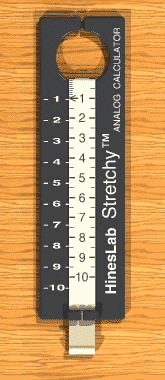# Stretchy Calculator

Math teaching aidThis Stretchy analog calculator helps the student understand why the answers change in the way they do.

Digital calculators provide answers but no insight into how the answers are derived.

The Stretchy calculator has a numerical scale printed on a rubber band which is pulled while holding the calculator by the large hole.  The number “1” (and arrow) on the rubber band is the index, and is important in almost every calculation.

The calculator’s accuracy is limited to 1-2 decimal places; however, excels at teaching and illustrating the proportional expansion of the answers.

 Stretchy calculator operation:MULTIPLICATION:  To multiply X x Y, pull the rubber band until the index (the number “1”) is next to the X value on th,e fixed scale.  Opposite the Y value on the rubber band, read the answer.  The animation illustrates two examples, 2 x 4 = 8, and 3 x 2 = 6. DIVISION:  To divide, X÷Y, set the Y value on the rubber band next to the X value on the fixed scale.  Read the answer, on the fixed scale, next to the index (the number “1”).  Example: 8 ÷ 4.  Set the 4 on the rubber band next to the 8 on the fixed scale.  The index on the rubber band is pointing to the answer, 2, on the fixed scale.   RATIOS: X/Y:  Example, 2/5, stretch the rubber band until the 2 on the rubber band is adjacent to the 5 on the fixed scale, then adjacent to the 1 on the fixed scale, read 4/10 on the rubber band (or for more accuracy, adjacent to the 10 on the fixed scale, read 4 on the rubber band, and mentally shift the decimal to yield 0.4). PROPORTIONS:  (X/Y) x N: Example: (2/3) x N.  Stretch the rubber band to make the 2 on the rubber band line up with the 3 on the fixed scale.  Then, using the numbers on the fixed scale, read to the right to find 2/3 the value of any number on the fixed scale.  Ex.: (2/3)3 = 2;  (2/3)4 = 2-2/3;  (2/3)6 = 4;  (2/3)9 = 6, etc. SQUARES:  X2:  To square X, set the index (“1”) to the value of X on the fixed scale.  Adjacent to the value of X on the rubber band, read the answer on the fixed scale.  Example: 3 squared: set the index on the rubber band to 3 on the fixed scale, and, read the answer 9 on the fixed scale. SQUARE ROOTS:  The square root of X, stretch the rubber band until the same value on the fixed scale (adjacent to the index on the rubber band), and which is on the rubber band, lines up adjacent to the value of X on the fixed scale.  Example: to find the square root of 9, stretch the rubber band until you find that the value on the fixed scale lines up with the index on the rubber band at the same time the same value, 3 on the rubber band, lines up with the 9 on the fixed scale.  The square root of 9 = 3. DECIMAL EQUIVALENTS:  To convert X/Y, set X on the rubber band adjacent to Y on the fixed scale, then adjacent to “1” on the fixed scale read the decimal equivalent on the rubber band.  Example: 2/3: set the 2 on the rubber band adjacent to 3 on the fixed scale.  Adjacent to 1 on the fixed scale (or “10” for more accuracy) read 0.66 on the rubber band. FRACTIONAL EQUIVALENTS:  To convert a number in decimal form, set the number on the rubber band adjacent the 1 or 10 on the fixed scale, and find the pair of numbers that most nearly align with each other.  Notice, for example, that when calculating the fractional equivalent of 0.333, the answers of 3/9, 2/6 and 1/3 are all equivalent.

HinesLab is actively seeking licensees to commercialize this technology.  This is not a product being offered for sale to end users.  To discuss licensing, please contact Steve Hines at:

HinesLab

USA

phone:  818-507-5812

email: Steve@HinesLab.com